Courses

# Test: Circuit Analysis in the S-Domain

## 20 Questions MCQ Test RRB JE for Electronics & Communication Engineering | Test: Circuit Analysis in the S-Domain

Description
This mock test of Test: Circuit Analysis in the S-Domain for Railways helps you for every Railways entrance exam. This contains 20 Multiple Choice Questions for Railways Test: Circuit Analysis in the S-Domain (mcq) to study with solutions a complete question bank. The solved questions answers in this Test: Circuit Analysis in the S-Domain quiz give you a good mix of easy questions and tough questions. Railways students definitely take this Test: Circuit Analysis in the S-Domain exercise for a better result in the exam. You can find other Test: Circuit Analysis in the S-Domain extra questions, long questions & short questions for Railways on EduRev as well by searching above.
QUESTION: 1

### The ratio of the transfer function Io/Is is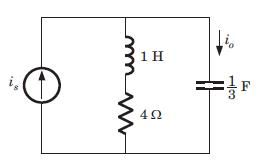Solution:

Io/Is = (s + 4)/ (s + 4 + 3/s) = s(s + 4)/(s + 1)(s + 3).

QUESTION: 2

### The voltage across 200 μF capacitor is given by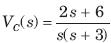The steady state voltage across capacitor is

Solution: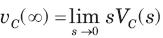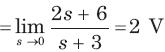QUESTION: 3

### The transformed voltage across the 60 μF capacitor is given by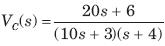The initial current through capacitor is

Solution: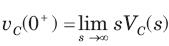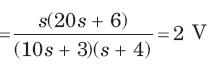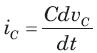⇒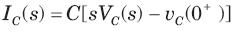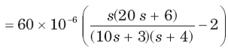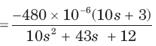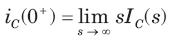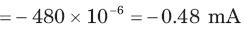QUESTION: 4

The current through an 4 H inductor is given by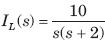The initial voltage across inductor is

Solution: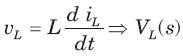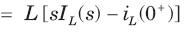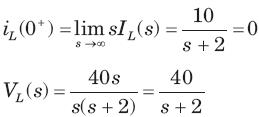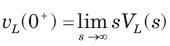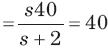QUESTION: 5

The amplifier network shown in fig.is stable if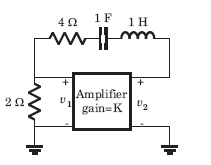Solution: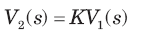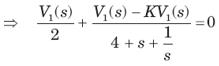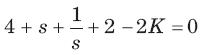⇒ s2 + (6 - 2K) s + 1 = 0
(6 - 2K) > 0  ⇒ K < 3

QUESTION: 6

The network shown in fig. is stable if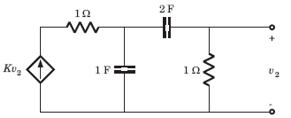Solution:

Let v1 be the node voltage of middle node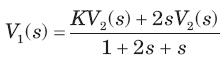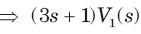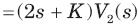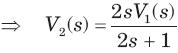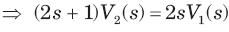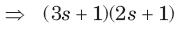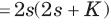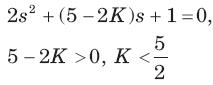QUESTION: 7

A circuit has a transfer function with a pole s = -4 and a zero which may be adjusted in position as s= -a The response of this system to a step input has a term of form Ke -4t. The K will be (H= scale factor)

Solution: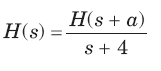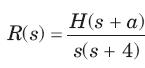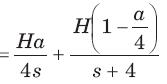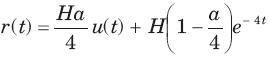QUESTION: 8

A circuit has input vin (t) = cos 2t u(t) V and output io (t) = 2sin 2t u(t) A. The circuit had no internal stored energy at t = 0. The admittance transfer function is

Solution: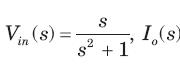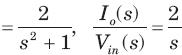QUESTION: 9

A two terminal network consists of a coil having an inductance L and resistance R shunted by a capacitor C. The poles of the driving point impedance function Z of this network are at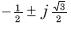and zero at -1. If Z(0) =1the value of R, L, C are

Solution: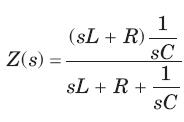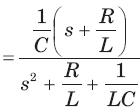QUESTION: 10

The current response of a network to a unit step input is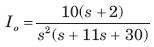The response is

Solution:

The characteristic equation is  s2 (s2 + 11s + 30) = 0 ⇒ s2 (s + 6)(s + 5) = 0
s = -6, -5, Being real and unequal, it is overdamped.

QUESTION: 11

The circuit is shown in fig.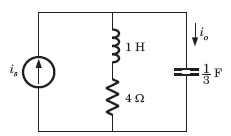The current ratio transfer function Io/IS  IS

Solution: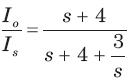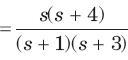QUESTION: 12

The circuit is shown in fig.The response is

Solution:

The characteristic equation is (s+1 (s+3) = 0. Being real and unequal root, it is overdamped response.

QUESTION: 13

The circuit is shown in fig.If input is is 2u(t) A, the output current io is

Solution: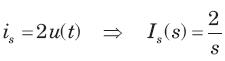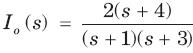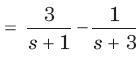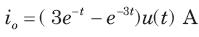QUESTION: 14

In the network of Fig. , all initial condition are zero. The damping exhibited by the network is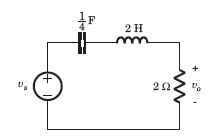Solution: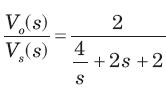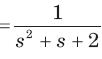The roots are imaginary so network is underdamped

QUESTION: 15

The voltage response of a network to a unit step input is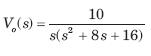The response is

Solution:

The characteristic equation is s(s2 + 8s + 16) = 0 , (s + 4)2 = 0, s = -4, -4

Being real and repeated root, it is critically damped.

QUESTION: 16

The response of an initially relaxed circuit to a signal vs is e-2t u(t). If the signal is changed to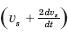, the response would be

Solution: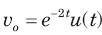⇒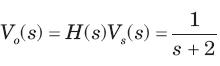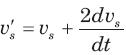⇒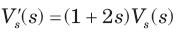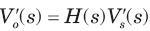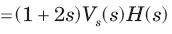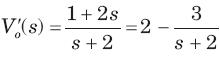⇒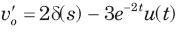QUESTION: 17

Consider the following statements in the circuit shown in fig.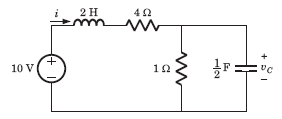1. It is a first order circuit with steady state value of vC= 10/3, i = 5/3A

2. It is a second order circuit with steady state of v= 2 V , i = 2 A

3. The network function V(s)/I(s)has one pole.

4. The network function V(s)/I(s) has two poles.

Solution:

It is a second order circuit. In steady state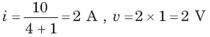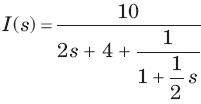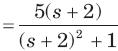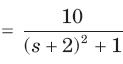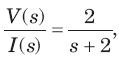It has one pole at s = -2

QUESTION: 18

The network function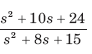represent a

Solution: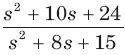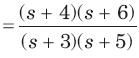The singularity near to origin is pole. So it may be RC impedance or RL admittance function.

QUESTION: 19

The network function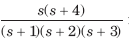represents an

Solution:

Poles and zero does not interlace on negative real axis so it is not a immittance function

QUESTION: 20

The network function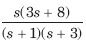represents an

Solution:

The singularity nearest to origin is a zero. So it may be RL impedance or RC admittance function. Because of (D) option it is required to check that it is a valid RC admittance function. The poles and zeros interlace along the negative real axis. The residues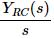are real and positive.